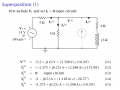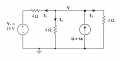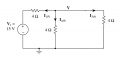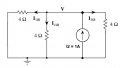# Why is the Current Phasor I1 (-2.375+j0.25)A instead of (2.375-j0.25)A

#### Mojo Pin__

Joined Apr 13, 2019
83
We are learning about superposition and I understand the basic concept, I have been calculating the currents and voltages marked out on this page, why would the signs be opposite for I1, is it the direction of current flow? I thought if the current source has become open circuit the current would have to flow into the node from V through the first 4 ohm resistor. Can anyone advise how best to understand this type of problem,

Thanks,

Keith#### Jony130

Joined Feb 17, 2009
5,457
Did you notice that the current I1 is flowing in the "opposite" direction (assumed "positive" direction) to the I1(1) current "forced by" V1 voltage source alone?

•Mojo Pin__

#### Mojo Pin__

Joined Apr 13, 2019
83
Did you notice that the current I1 is flowing in the "opposite" direction (assumed "positive" direction) to the I1(1) current "forced by" V1 voltage source alone?
In this picture I think the I1(1) arrow is the wrong way round if the current source has been open-circuited. Would you agree with that?

I'm not sure I understand what you mean, I would expect the current to flow clockwise from the voltage source through the first resistor and then branch off in to the parallel paths. So I1(1) would be positive current flow and I3(1) and I4(1) would be negative according to KCL.

Am I right in thinking that with phasors if you flip the signs the it just means the opposite direction? I feel like I'm missing some critical knowledge all the time with electronics, my brain is fried today.

#### Jony130

Joined Feb 17, 2009
5,457
In this picture I think the I1(1) arrow is the wrong way round if the current source has been open-circuited. Would you agree with that?

I'm not sure I understand what you mean, I would expect the current to flow clockwise from the voltage source through the first resistor and then branch off in to the parallel paths. So I1(1) would be positive current flow and I3(1) and I4(1) would be negative according to KCL.
What was the originally assumed (positive) direction of a I1 current?
We have the same situation in DC when we are dealing with superposition.We open the current source and we have:I_1(1) = - 15V/6Ω = -2.5A (do you know why I add a minus sign? )
I_3(1) = 1.25A
I_4(1) = 1.25A
V(1) = 5V

Next, we turn off the Voltage source.I_1(2) = 0.333A
I_4(2) = 0.333A
I_3(2) = 0.333A
V(2) = 1.333V

Therefore the final solution is

I_1 = I_1(1) + I_1(2) = -2.5A + 0.333A = 2.1667A
I_3 = I_3(1) + I_3(2) = 1.25A + 0.333A = 1.583A
I_4 = I_4(1) + I_4(2) = 1.25A + 0.333A = 1.583A
V = V(1) + V(2) = 6.333V

Do you see the similarities?

Am I right in thinking that with phasors if you flip the signs the it just means the opposite direction?
Yes, you are right.

•Mojo Pin__

#### WBahn

Joined Mar 31, 2012
29,175
In this picture I think the I1(1) arrow is the wrong way round if the current source has been open-circuited. Would you agree with that?

I'm not sure I understand what you mean, I would expect the current to flow clockwise from the voltage source through the first resistor and then branch off in to the parallel paths. So I1(1) would be positive current flow and I3(1) and I4(1) would be negative according to KCL.

Am I right in thinking that with phasors if you flip the signs the it just means the opposite direction? I feel like I'm missing some critical knowledge all the time with electronics, my brain is fried today.
When applying superposition, you need to be very systematic. On the diagram that has everything, assign all of your reference voltages and currents. You can pick whatever polarity you want for them -- flip a coin if you want to -- but once you assign them you should stick with them. Then for each of the individual analyses, you stick with the defined voltages and currents, including the polarities. That way, at the end, you can simply add them all up. If you start going through and changing the directions of some of the voltages and currents in some of the analyses then you have to be extremely careful to keep track of which ones you need to add and which ones you need to subtract to get the total result -- that's a recipe for mistakes that are extremely easy to make and difficult to catch.

#### Mojo Pin__

Joined Apr 13, 2019
83
Thank you for your explanation, it is starting to become a bit clearer.

I_1(1) = - 15V/6Ω = -2.5A (do you know why I add a minus sign? )
Is it because the voltage polarity opposes the flow of current indicated by I_(1) ?

Do you see the similarities?
I do, thank you, i tried to do this with DC earlier just to see if it would be a bit easier but I just got more confused. I'll start it over again from the beginning.

#### Mojo Pin__

Joined Apr 13, 2019
83
When applying superposition, you need to be very systematic. On the diagram that has everything, assign all of your reference voltages and currents. You can pick whatever polarity you want for them -- flip a coin if you want to -- but once you assign them you should stick with them. Then for each of the individual analyses, you stick with the defined voltages and currents, including the polarities. That way, at the end, you can simply add them all up. If you start going through and changing the directions of some of the voltages and currents in some of the analyses then you have to be extremely careful to keep track of which ones you need to add and which ones you need to subtract to get the total result -- that's a recipe for mistakes that are extremely easy to make and difficult to catch.
I will try to be more systematic, i find these problems a bit daunting at the start (and these are the basic circuits!), easy for me to get confused but I will take my time and do as you say. Thanks again for all your help.

#### Jony130

Joined Feb 17, 2009
5,457
Is it because the voltage polarity opposes the flow of current indicated by I_(1) ?
Yes, exactly. The assumed I_1 direction is opposed to the direction of the current flow "generated" by a voltage source alone.

•Mojo Pin__

#### Mojo Pin__

Joined Apr 13, 2019
83
Thats great, i think I'm good for now, i just need to practice more of these types of questions. Thanks for your help!

#### MrAl

Joined Jun 17, 2014
10,617
We are learning about superposition and I understand the basic concept, I have been calculating the currents and voltages marked out on this page, why would the signs be opposite for I1, is it the direction of current flow? I thought if the current source has become open circuit the current would have to flow into the node from V through the first 4 ohm resistor. Can anyone advise how best to understand this type of problem,

Thanks,

Keith
View attachment 212677
Hi,

When i do a quick analysis of the current through that 4 Ohm resistor connected to V1 i calculate the current in an "assumed" direction of left to right. I get the result:
2.375-0.25*j

But then when i look at the diagram i see they wanted to use an assumed direction of just the opposite of what i assumed, they wanted to assume right to left for I1, so i have to make my calculated current negative which makes it come out to:
-(2.375-0.25*j)

which of course after distributing the minus sign comes out to:
-2.375+0.25*j

So what we had to deal with here was the 'assumed' direction of current which was decided beforehand with this particular problem. In another problem it may just be stated as just the opposite and in that case i would not have had to change anything after my first calculation.

•Mojo Pin__

#### Mojo Pin__

Joined Apr 13, 2019
83
Hi,

When i do a quick analysis of the current through that 4 Ohm resistor connected to V1 i calculate the current in an "assumed" direction of left to right. I get the result:
2.375-0.25*j

But then when i look at the diagram i see they wanted to use an assumed direction of just the opposite of what i assumed, they wanted to assume right to left for I1, so i have to make my calculated current negative which makes it come out to:
-(2.375-0.25*j)

which of course after distributing the minus sign comes out to:
-2.375+0.25*j

So what we had to deal with here was the 'assumed' direction of current which was decided beforehand with this particular problem. In another problem it may just be stated as just the opposite and in that case i would not have had to change anything after my first calculation.
I found that to be the case as well, thank you for responding to my problem.

#### Mojo Pin__

Joined Apr 13, 2019
83
I don't really understand how I'm supposed to calculate V(1), let alone how the professor managed to calculate it first, i got V(1) =10.25 +j6.125

I found the total impedance of the circuit with I2(1) =0

Ztotal= 456/73 + j48/73

Then I1(1) = -15/Ztotal

So I1(1) = -2.375 + j0.25

From this I know that I1(1) = I3(1) + I4(1)

I don't know where to go from here, I thought I could say that

I3+4(1) = 2.375-j0.25

So V(1) = (2.375-j0.25)(4+j3) = 10.25 + j6.125

I also tried using voltage divider

V(1) = (15(4+j3))/(4+4+j3) = 8.42 + j2.5 which is different and also wrong.

Any advice on what I'm doing wrong here?

Thanks!

I have spotted an error in my working should be using (164/73)+j(48/73) for the parallel impedance not 4+j3.
Working through the problem again, apologies.

I got it! both the voltage divider and KCL got me the correct answer, I just messed up the numbers when I was dealing with the parallel impedance, thanks for helping me wit this problem, I feel like I understand it all a bit better now.

Last edited:

#### Jony130

Joined Feb 17, 2009
5,457
From this I know that I1(1) = I3(1) + I4(1)

I don't know where to go from here, I thought I could say that

I3+4(1) = 2.375-j0.25

So V(1) = (2.375-j0.25)(4+j3) = 10.25 + j6.125
This is wrong because the total impedance of "lower part" of the circuit is 4Ω|| (4Ω + j3Ω) = 164/73 + j48/73 not (4Ω + j3Ω), you cannot ignore 4 ohms resistor in parallel with resistor + inductor (4Ω + j3Ω)

Therefore the voltage is V(1) = I_tot*Z = (2.375 - j0.25) * (164/73 + j48/73) = 5.5 + j1

I also tried using voltage divider

V(1) = (15(4+j3))/(4+4+j3) = 8.42 + j2.5 which is different and also wrong.

Any advice on what I'm doing wrong here?
Again, why did you ignore 4 ohm's resistor in parallel with 4 ohm's resistor + inductor?

V = V1 * 4Ω|| (4Ω + j3Ω)/(4Ω + 4Ω|| (4Ω + j3Ω)) = 15 * (164/73 + j48/73)/(4 +164/73 + j48/73 ) = (5.5 + j1)V

•Mojo Pin__

#### Mojo Pin__

Joined Apr 13, 2019
83
This is wrong because the total impedance of "lower part" of the circuit is 4Ω|| (4Ω + j3Ω) = 164/73 + j48/73 not (4Ω + j3Ω), you cannot ignore 4 ohms resistor in parallel with resistor + inductor (4Ω + j3Ω)

Therefore the voltage is V(1) = I_tot*Z = (2.375 - j0.25) * (164/73 + j48/73) = 5.5 + j1

Again, why did you ignore 4 ohm's resistor in parallel with 4 ohm's resistor + inductor?

V = V1 * 4Ω|| (4Ω + j3Ω)/(4Ω + 4Ω|| (4Ω + j3Ω)) = 15 * (164/73 + j48/73)/(4 +164/73 + j48/73 ) = (5.5 + j1)V
yes! thank you for responding, I realised this and went through the question again with this correction and I arrived at the correct answer. Thank you so much for your help, I often make simple errors like this but I am getting better through practice